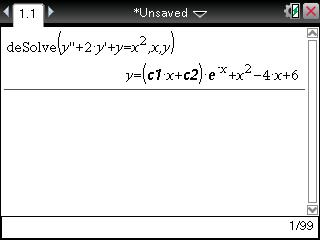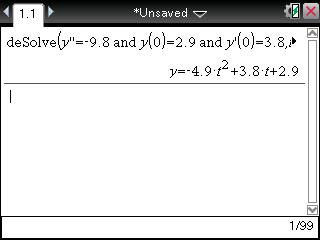# Knowledge Base

## Solution 29650: Solving Second Order Differential Equations Using the TI-Nspire™ CAS Family Products.

### How do I solve a second order differential equation using the TI-Nspire CAS family products?

First and second order differential equations can be solved by using the deSolve( command.

For example: First Order Equation

Solve the equation y''+2y'+y=x2.

1) Press [Menu] [4: Calculus] [D: Differential Equation Solver]

Please Note: To get ", press the [?!>] key and select ' from the template. Select ' twice instead of selecting ".

2) Enter [y] ['] ['] [+]  [y] ['] [+] [y] [=] [x] [^]  [,] [x] [,] y]
3) Press [ENTER] to display the answer. The variables c1, c2, etc are constants.For example: Second Order Equation

A ball is tossed straight up from an initial height of 0.29 meters and with an initial velocity of 3.8m/s. Solve the second order differential equation below to model the height of the ball over time:

y"=-9.8, y(0)=.29, y'(0)=3.8

1) Press [Menu] [4: Calculus] [D: Differential Equation Solver]